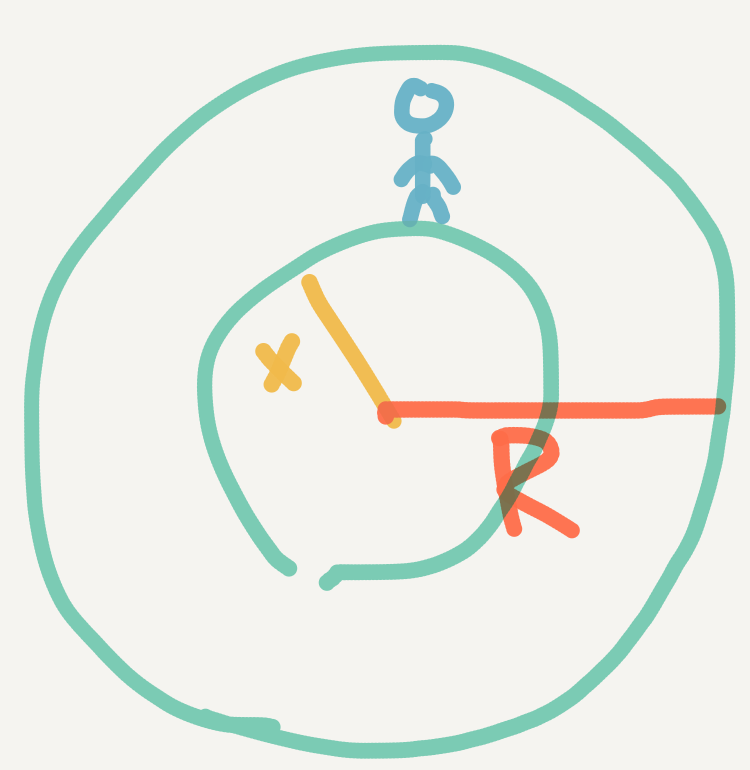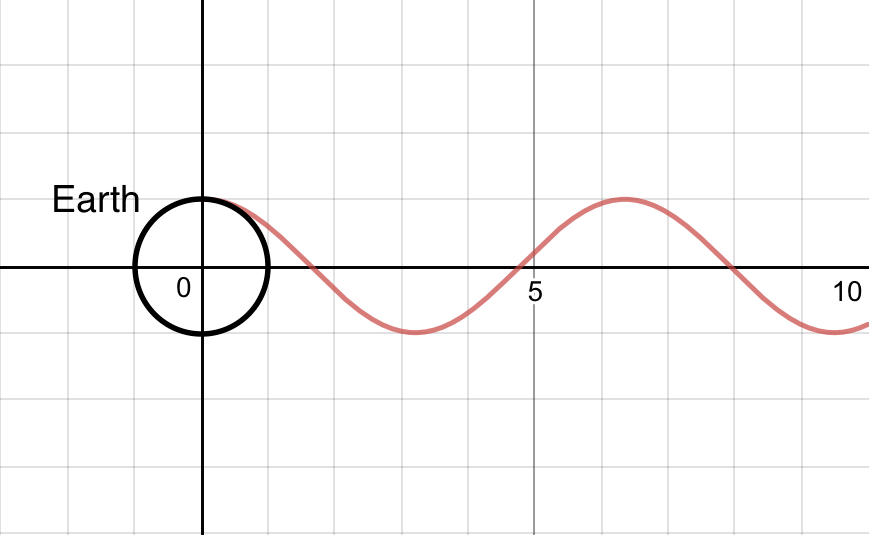The Earth has a diameter of approximately 12742000 meters. Most people of course wouldn't travel that far, but what if you did? How fast can you get across with nothing but yourself? That's essentially what people have asked in the form of the question: How long will it take to fall through the center of the Earth?

SPOLIER WARINING:
THIS WARNING IS TO INDICATE ANY WORD FOLLOWING THIS MESSAGE WILL BE A PART OF THE SOLUTION TO THIS PROBLEM. HIGHLY ENCOURAGE THE ATTEMPT TO SOLVE THIS PROBLEM.
YOU HAVE BEEN WARNED...

Following our standard procedure, let's list all the givens:
$The\,radius\,of\,the\,Earth is R = 6,371,000$ meters

$The\,Force\,of\,Gravity\,is\,F = \large \frac{G m M}{r^2}$

Where...
$G = Gravity$
$m = Mass\,of\,Object_1\, (in\,this\,case,\,us)$
$M = Mass\,of\,Object_2\,(in\,this\,case,\,Earth)$
$r = the\,distance\,between\,m\,and\,M.$

So now we are just trying to find as many values or expressions to variables within that eqauation of force. We can leave $m$ as is, becuase that's the mass of our human/us. So what we really need is $r$ and $M$.One thing we have to worry about though, is that as we fall $r$ will change. As we fall we will get clsoer to Earth's center of mass, eventually pass it, and then get farther from it. So we will call our current distance relative to Earth's center of mass as $x$. And what's great about this, if we are any distance into our fall, we can just ignore any mass above us. Using the diagram as an example, if we are $R-x$ deep into our fall, we can ignore any mass of Earth contained between $R$ and $x$. Some of you may think, "But wait! Wouldn't the mass above us have it's own force of gravity acting upon you, and therefore slowing you down as you fall?" The answer is technically yes, but that all balances out with the mass below you and to the side of you. All these forces cancel out, making it not affect you at all. So, all we really care about is the amount of mass below us, and the distance between us and the Earth's center of mass (which would be the radius $x$ as we have been discussing). So we have one variable filled.

$F = \large \frac{G m M}{x^2}$

Now we need $M$. The formula for mass is $M = volume \times density$. The volume of the Earth $= \frac{4 \pi x^3}{3}$ (we are using $x$ again as the mass affecting us changes over our fall). And we can represent density with $\rho$. So the $M$ equals:

$\large \frac{4 \pi \rho x^3}{3}$

Putting this all together, the force of gravity acting upon us during this fall equals:

$F = \large \frac{4 \pi G m \rho x^3}{3 x^2}$

$= \large \frac{4 \pi G m \rho}{3} x$

If we let $\frac{4 \pi G m \rho}{3} =$ say, v, we get $F = -v x$. It's negative because we are falling first. This is actually an oscillating system. To represent this, I've made a mock graph to show how gravity affects us over time starting from the top of "Earth". The graph is just a representation.If the x-axis is time, and we fell from the top of Earth (and there is NO air resistence), as you can see, we would just continuously bounce back and forth between the top and bottom of the Earth. Now we need to find the period of our oscillating system. The period is the time it takes for one cycle to be completed. To be more precise, we need half of the period. That is because one cycle (in this case) is falling all the way down, and coming all the way back. We only want the time it takes to fall down, so that's why the half.

The eqauation for the period of a simple oscillating system (also called a harmonic motion) is:

$P = 2 \pi \sqrt {\large \frac {m}{k}}$

The variable representation is that $k$ is our oscillating system, and $m$ is our mass. But since we want half of that, so therefor time to fall through the Earth is...

$Time = \pi \sqrt {\large \frac {m}{k}}$

Doing some substitution...

$Time = \pi \sqrt {\large \frac {m}{\large \frac{4 \pi G m \rho}{3}}}$

$= \pi \sqrt {\large \frac {3 m }{4 \pi G m \rho}}$

$= \sqrt {\large \frac {3 m \pi^2}{4 \pi G m \rho}}$

$= \sqrt {\large \frac {3 \pi}{4 G \rho}}$

Now all we need to do is put in $G$ as the Gravitational Constant, and $\rho$ as the density ($\rho = \frac{mass}{volume}$) of Earth (I did some Googling...)!

$Time = \sqrt {\large \frac {3 \pi}{4 \cdot 6.67408 \cdot 10^{-11} \cdot m^3 \cdot kg^{-1} \cdot s^{-2} \cdot \frac{5.972 \cdot 10^{24}\, kg}{\frac{4 \pi \cdot 6371000^3}{3} \, m^3} }}$

This looks bad. Let's clean it up.

$= \sqrt {\large \frac {3 \pi}{4 \cdot 6.67408 \cdot 10^{-11} \cdot m^3 \cdot kg^{-1} \cdot s^{-2} \cdot \frac{5.972 \cdot 10^{24} \cdot 3 \, kg}{4 \pi \cdot 6371000^3 \, m^3} }}$

$= \sqrt {\large \frac {3 \pi}{4 \cdot 6.67408 \cdot 10^{-11} \cdot s^{-2} \cdot \frac{5.972 \cdot 10^{24} \cdot 3}{4 \pi \cdot 6371000^3} }}$

$= \sqrt {\large \frac {3 \pi \cdot s^{2}}{4 \cdot 6.67408 \cdot 10^{-11} \cdot \frac{5.972 \cdot 10^{24} \cdot 3}{4 \pi \cdot 6371000^3} }}$

$= s \sqrt {\large \frac {3 \pi}{4 \cdot 6.67408 \cdot 10^{-11} \cdot \frac{5.972 \cdot 10^{24} \cdot 3}{4 \pi \cdot 6371000^3} }}$

So, I don't know about you, but when I have something like this, I just straight up put it into Wolfram Alpha , or a similar calculator, as I am just lazy and it's a pain to evaluate. So, letting it be computed by the calculator...

$Time = s \sqrt {\large \frac {3 \pi}{4 \cdot 6.67408 \cdot 10^{-11} \cdot \frac{5.972 \cdot 10^{24} \cdot 3}{4 \pi \cdot 6371000^3} }}$

$\large = 2530.5\,seconds$

This, funnily enough is also the answer to the universe and all of its questions. $2530.5\,seconds = 42\,minutes\,(+10.5\,seconds)$. Quite a coincidence if I say so!

Now what's great about our equation we used ($= \sqrt {\frac {3 \pi}{4 G \rho}}$), it's quite easy to apply to other objects, as most of it is constant! 3, is well, a constant. So is 4. $\pi$ has been universally agreed upon for its value. And as far as we can tell in the universe, the Gravitational Constant is true. The only thing that determines the fall length is the density. So you could have two planets, one with $x$ as its radius, and the other as $100 x$. If the are just as dense as one another, you will fall through them (across the diameter) in the same time.

Now just as a random fact that I thought was amusing, was the top speed you would attain. We know that acceleration due to gravity on Earth is $\frac{9.807\,m}{s^2}$. The top speed would be when you reach the center of the Earth, which is 6,371,000 meters from the surface (aka, the radius of Earth). Using this, we can calculate the speed at which we would be at in meters per second at the center. Just to be sure, we can calculate acceleration due to gravity, using our original formula, where we ignore our mass: $g = \frac{G \cdot M_{Earth}}{R^2}$

$g = \large \frac{G \cdot M_{Earth} }{R^2}$

Substituting everything we have...

$g = \frac{6.67408 \cdot 10^{-11} \cdot m^3 \cdot kg^{-1} \cdot s^{-2} \cdot 5.972 \cdot 10^{24}\, kg }{6371000^2\,m^2}$

$g = \frac{6.67408 \cdot 5.972 \cdot 10^{13} \cdot m}{6371000^2 \cdot s^2}$

Thanks to a calculator...

$\approx \large\frac{9.82m}{s^2}$

Of course this is not the same as what others have put on the internet, values will differ from here to there. I trust the value of $\frac{9.807m}{s^2}, as I think there values they used to calculate it would be more accurate. Back to the top speed now.$g = \large \frac{9.807\,m}{s^2} = \large \frac{9.807 \cdot 6371000}{s^2} = \large \frac{\sqrt{9.807 \cdot 6371000}}{s}\approx \large \frac{7904.454251 m}{s}$Which is equivalent to...$\approx \large \frac{28456.035304\,km}{hour}\approx \large \frac{17681.760583\,miles}{hour}$That's about 23.23 times the speed of sound! This literally means you can't yell during this fall, as you would be going literally faster than the time it takes to vibrate the air around you. It will be a silent fall. That is, if there was air, and the terminal velcoity of a human wasn't$\frac{53m}{s}$. So that would be it for this post! We found out we can cross Earth in under 45 minutes, and break the sound barrier 23 times over! I plan on following it up with another post showing how you can use integration to find the time to fall through Earth (and that equation to find the period of an oscillating system/simple harmonic motion that kind of came out of nowhere. The$2 \pi \sqrt{\frac{m}{k}}\$), and to show some other cool properties and interesting things about falling, pendulums, and oscillating systems in general.

Now here's an extra challenge for you: How long will it take to fall through Earth, 500 kilometers above the surface?